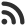Feed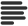Articles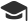Tutorials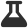Lab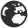CompaniesLeaderboard
 DG Student at BIT Mesra March 31, 2020, 10:25 p.m. ⋅ 751 views

# Babylonian method to find the square root

Babylonian method to find the square root is based on Newton–Raphson method.

We start form y=1 and x = number and then we find approximate root by finding average x and y. With each iteration, we update value of y = number / x.

Algorithm

``````Begin
x := number
y := 1
precision := 0.001
while error > precision, do
x := (x+y) / 2
y := number / x
done
return x
End``````

Implementation

``````#include<bits/stdc++.h>
using namespace std;

float bbln_sqrt(float number) {
float x = number, y = 1;              // let's say sqrt of number is 1
float precision = 0.001;           // Considering precision of 0.001

while(abs(x - y)/abs(x) > precision) {
x = (x + y)/2;
y = number/x;
}
return x;
}

int main() {
int n;
cout << "Enter Number ";
cin >> n;
cout << "SQRT of " << n <<" is: " << bbln_sqrt(n);
}``````
``````Enter Number 78
SQRT of 78 is: 8.83177``````

Events

Nov. 28, 2018, 5:30 p.m.

Python from zero to hero

place Delhi

Aug. 13, 2018, 5:30 p.m.

Python from zero to hero

place Bangalore ( HackersFriend office BTM Layout)